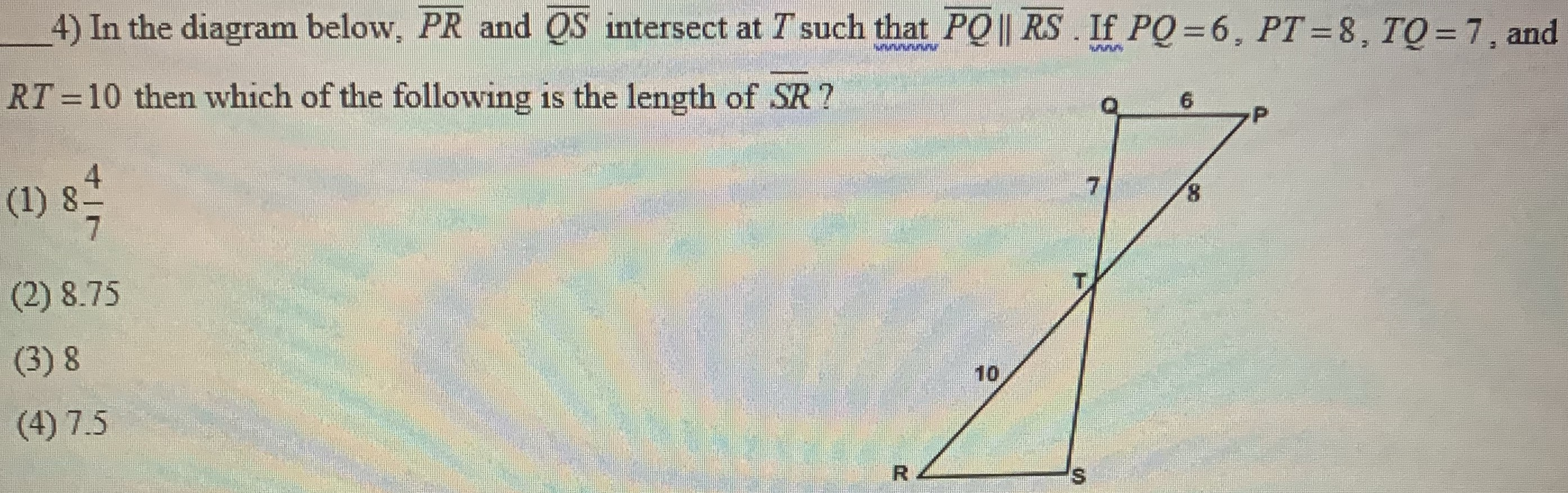¿Todavía tienes preguntas de matemáticas?

Pregunte a nuestros tutores expertos
Geometry
QuestionIn the diagram below,$$\overline{PR}$$ and $$\overline{QS}$$ intersect at $$T$$ such that $$\overline{PQ} \left | \right |\overline{RS}$$. If $$PQ=6,PT=8,TQ=7$$, and $$RT=10$$ then which of the following is the length of $$\overline{SR}$$?

$$7.5$$